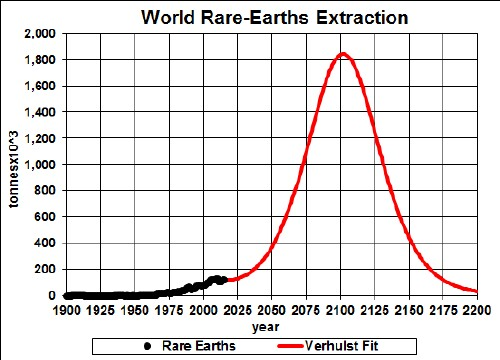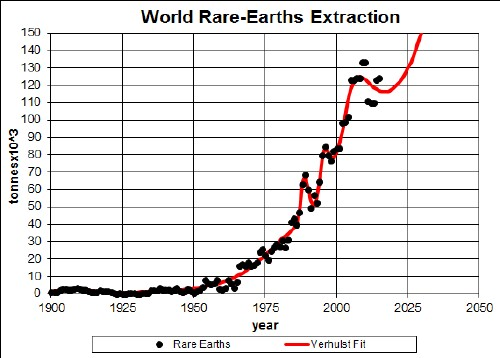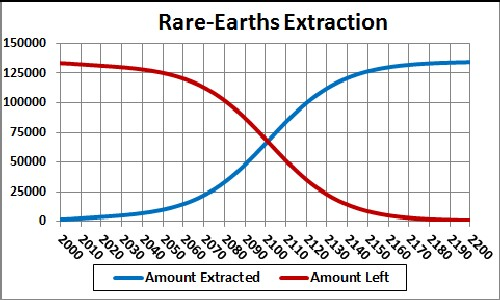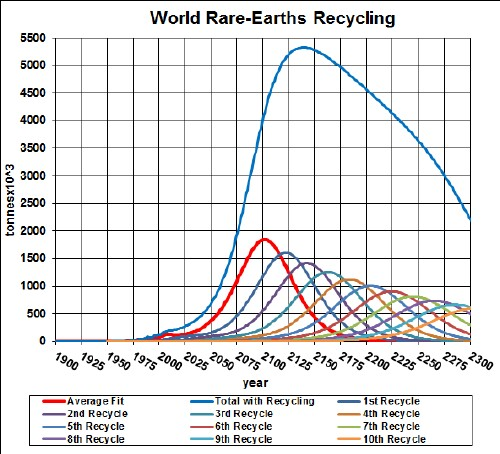# Rare-Earths Depletion Including Recycling

The fifteen Rare Earths (Lantham, Cerium, Praseodymium, Neodymium, Promethium, Samarium, Europium, Gadolinium, Terbium, Dysprosium, Holmium, Erbium, Thulium, Ytterbium & Lutetium) are used to make many modern technological devices, including magnets. The graph below shows the rare.earths extraction data for the world and a Verhulst functions fit to the data in order to extrapolate into the future.Rare-Earths extraction rate for the world and a Verhulst functions fit to the data.The red curve is a fit with total amount to be extracted of which incorporates the latest reserves estimate (130 x 106 tonnes).

It appears that world-rare-earths extraction will peak at ~2100.

Taking an average extraction curve of the two fits, the crossover point at year ~2100 when the amount extracted is equal to the amount left to be extracted is shown here:## Recycling

Rare earths, especially the rare earths used in magnets, is recycled to a high degree. Assume that:

• The rare-earths-extraction curve is the average of the two curves given above.
• Recycling of rare earths follows a hyperbolic tangent curve from 50% to 95% recycling with a break point of year 1980 and width 25 years.
• The recycling is delayed by a Gaussian curve peaking at a delay of 20 years and a width of 10 years.

The effective rare earths available for making items after the first ten recycling cycles is shown in the following graph, along with the effective rare earths available for each cycle:The equation for a recyling cycle is,

where Ei is the amount available from the previous cycle. Here is an example of the Excel coding:

{=((\$J\$2+\$I\$2)/2+((\$J\$2-\$I\$2)/2)*TANH((A27-\$K\$2)/\$L\$2))*SUM(\$I\$27:I27*(EXP(-1*((A27-\$A\$27:A27-\$N\$2)/\$O\$2)^2/2))/\$O\$2/SQRT(2*PI()))} (The curly bracket surrounding the term makes it into an array; it must be entered by holding down the SHIFT & CTRL keys while pressing the ENTER key.)

Of course, the recycling could be extended to more cycles, skewing the curve further into the future. However, the peak and fall off after it will not change because further cycles are essentally zero in that time region.

Thus, under the assumptions given above, the effective amount of rare earths available for making items peaks at about 2070 and falls off rapidly after that. Humans will have taken concentrated rare earths deposits and scattered them across the surface of the earth.

The Excel spreadsheet is set up to make it easy to calculate with different recycling assumptions.

Minerals Depletion